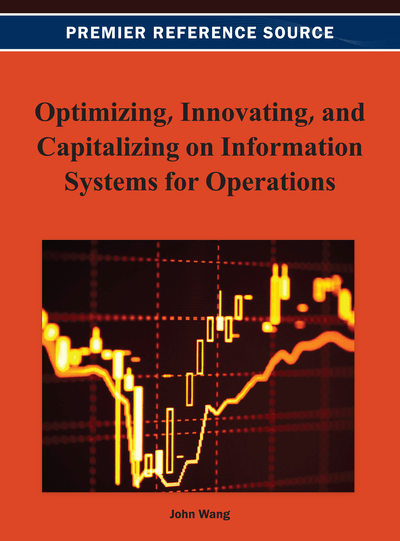# Data Envelopment Analysis with Fuzzy Parameters: An Interactive Approach

DOI: 10.4018/978-1-4666-2925-7.ch006
OnDemand:
(Individual Chapters)
Available
\$37.50
No Current Special Offers

## Abstract

Data envelopment analysis (DEA) is a methodology for measuring the relative efficiencies of a set of decision making units (DMUs) that use multiple inputs to produce multiple outputs. In the conventional DEA, all the data assume the form of specific numerical values. However, the observed values of the input and output data in real-life problems are sometimes imprecise or vague. Previous methods have not considered the preferences of the decision makers (DMs) in the evaluation process. This paper proposes an interactive evaluation process for measuring the relative efficiencies of a set of DMUs in fuzzy DEA with consideration of the DMs’ preferences. The authors construct a linear programming (LP) model with fuzzy parameters and calculate the fuzzy efficiency of the DMUs for different a levels. Then, the DM identifies his or her most preferred fuzzy goal for each DMU under consideration. A modified Yager index is used to develop a ranking order of the DMUs. This study allows the DMs to use their preferences or value judgments when evaluating the performance of the DMUs.
Chapter Preview
Top

## Fuzzy Dea Literature Review

In the conventional DEA, all the data assume the form of specific numerical values. However, the observed values of the input and output data in real-life problems are sometimes imprecise or vague. Imprecise evaluations may be the result of unquantifiable, incomplete and non obtainable information. The “Stochastic approach” and the “fuzzy approach” are two existence approaches for modeling uncertainty in the DEA literature. The stochastic approach involves specifying a probability distribution function (e.g., normal) for the error process (Sengupta, 1992). However, as pointed out by Sengupta (1992), the stochastic approach has two drawbacks associated with modeling the uncertainty in DEA problems:

• 1.

Small sample sizes in DEA make it difficult to use stochastic models, and

• 2.

In stochastic approaches, the DM is required to assume a specific error distribution (e.g., normal or exponential) to derive specific results. However, this assumption may not be realistic because on an a priori basis there is very little empirical evidence to choose one type of distribution over another.

## Complete Chapter List

Search this Book:
Reset